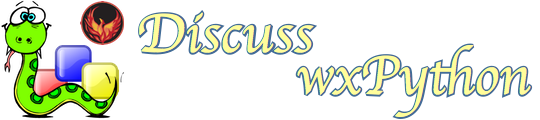# Issue with FloatCanvas Hit Color Generation on Ubuntu - event binding freezes program

Hi all!

When using wxPython 4.1.0 on Ubuntu 20.04.1 LTS, I stumbled upon a weird bug:
If I add multiple lines to a NavCanvas and bind an event to each, after some iterations the program starts lagging and in the end freezes until it throws the following exception:

``````Traceback (most recent call last):
File "/bug_test.py", line 92, in OnDraw
self.DrawLine(points, LnLbl, New_EndPt)
File "/bug_test.py", line 138, in DrawLine
new_line.Bind(FloatCanvas.EVT_FC_LEFT_DOWN, self.ObjLeftDown)
File "/env/lib/python3.8/site-packages/wx/lib/floatcanvas/FCObjects.py", line 236, in Bind
self.HitColor = next(self._Canvas.HitColorGenerator)
StopIteration
``````

It seems like the hit color generator is not able to generate a new hit color and iterates for ever, resulting in the freeze - if I understood the code correct.
However, using another platform, e.g. Windows, the problem does not occur. Someone else also experienced this bug and posted on stackoverflow: https://stackoverflow.com/questions/63320667/wxpython-floatcanvas-event-binding . According to this post, the problem also does not appear on Mint 19.

Does anyone know what to do about this bug? Thanks in advanceI’m posting the code example from the mentioned stackoverflow post which produces the bug on my machine:

``````import time
import string
import wx
from wx.lib.floatcanvas import NavCanvas, FloatCanvas
import wx.lib.colourdb

class InputForm(wx.Frame):
'''set up the form and draw axis'''

def __init__(self):
super(InputForm, self).__init__(None, wx.ID_ANY, title='Plot Lines', size=(1300, 830))

# set dictionary of points; key node letter, value tuple of point,
self.pts = {}
self.draw_repetitions = 0
# create the form level sizer
Main_Sizer = wx.BoxSizer(wx.HORIZONTAL)

# add the sizer for the left side widgets
sizerL = wx.BoxSizer(wx.VERTICAL)
# add the grid and then set it ot he left panel

btnsizer = wx.BoxSizer(wx.HORIZONTAL)
drw = wx.Button(self, -1, "Draw\nLines")
btnsizer.Add(drw, 0, wx.ALL | wx.ALIGN_CENTER, 5)

# bind the button events to handlers
self.Bind(wx.EVT_BUTTON, self.OnDraw, drw)

self.rght = NavCanvas.NavCanvas(self,
ProjectionFun=None,
Debug=0,
BackgroundColor="LIGHT GREY",
)
# self.Canvas = self.rght.Canvas

self.InitCanvas()

self.SetSizer(Main_Sizer)

def InitCanvas(self):
# add the x & y axis
self.Canvas = self.rght.Canvas
self.Canvas.ClearAll()
self.Canvas.AddLine([(0, 0), (0, 5)], LineWidth=2, LineColor='Yellow')
self.Canvas.AddLine([(0, 0), (5, 0)], LineWidth=2, LineColor='Green')
Color='blue',
Size=.5,
Width=None,
LineColor=None,
Family=wx.MODERN,
Position='tr',
Alignment='bottom',
InForeground=True)
# first Bind of node to EvtLeftDown
origin.Bind(FloatCanvas.EVT_FC_LEFT_DOWN,
lambda evt, selctEnd='Origin':
self.EvtLeftDown(evt, 'Origin'))

wx.CallAfter(self.Canvas.ZoomToBB)

def OnDraw(self, evt):
self.InitCanvas()
pts1 = (0, 0)

x = [i for i in range(5, 30, 2)]
y = x[::-1]
pts2 = [(x[i], y[i]) for i in range(0, len(x))]
alph = string.ascii_uppercase

LnLbls = [alph[i] for i in range(0, len(x))]

New_EndPt = True
n = 0
for pt in pts2:
points = []
points.append(pts1)
points.append(pt)
LnLbl = LnLbls[n]
New_EndPt = True
n += 1
self.DrawLine(points, LnLbl, New_EndPt)

def DrawLine(self, points, LnLbl, New_EndPt):
'''Draws the line object as specified in the VarifyData() function'''
self.draw_repetitions += 1
# label the end point of the line in lower case
if New_EndPt is True:
Color='black',
Size=.5,
Width=None,
LineColor=None,
Family=wx.MODERN,
Position='cc',
Alignment='bottom',
InForeground=True)

new_end.Bind(FloatCanvas.EVT_FC_LEFT_DOWN,
lambda evt, selctEnd=LnLbl.lower():
self.EvtLeftDown(evt, selctEnd))

# define the new line
# add the new line to the list of lines

# locate the center of the new line for the label location
lncntr = ((int(points) + int(points)) // 2,
(int(points) + int(points)) // 2)

# place the new line lable
Color='red',
Size=.5,
Width=None,
LineColor=None,
Family=wx.MODERN,
Position='tc',
Alignment='bottom',
InForeground=True)
new_line.Name = LnLbl

tic = time.perf_counter()
new_line.Bind(FloatCanvas.EVT_FC_LEFT_DOWN, self.ObjLeftDown)
toc = time.perf_counter()
print(f'time to execute BIND function for DrawLine line ', LnLbl, toc - tic)
print(f'Draw repetitions ', self.draw_repetitions)

# wx.CallAfter(self.Canvas.ZoomToBB)
self.Canvas.ZoomToBB()

def ObjLeftDown(self, object):
lbl = object.Name

if lbl == 'Origin':
self.Node(lbl)
print(dir(self.Node))
elif 65 <= ord(lbl) <= 90:
print('you have selected line ', lbl)
elif 97 <= ord(lbl) <= 122:
print('you have selected node ', lbl)

def EvtLeftDown(self, evt, lbl):
if lbl == 'Origin':
print('you have selected the origin')
elif 97 <= ord(lbl) <= 122:
print('you have selected node ', lbl)

#        try:
#            evt.Skip()
#        except:
#            pass

# Run the program
if __name__ == "__main__":
app = wx.App(False)
frame = InputForm()
frame.Center()
frame.Show()
app.MainLoop()
``````

I did, at least in some way, find out what caused my problem:
I was working in a virtual environment and installed wxpython using pip. That way wxpython was built from source on my machine and maybe that somehow caused this issue. Because when I left my virtual environment and installed the ubuntu package python3-wxgtk4.0 I could run the faulty program without any problems! So for now I can not use wxpython in an virtual environment, but at least I can use it.

So maybe this can help anyone facing the same problem.

One difference there: python3-wxgtk4.0 is wxPython 4.0.7 whereas you were
using 4.1.0 in your virtual environment. Despite the relatively small
version increase, there is a massive difference between 4.0.7 and 4.1.0.

Scott

You are right, didn’t even think about which version the package uses.

The code which seemed to cause the bug, _cycleidxs in https://github.com/wxWidgets/Phoenix/blob/89b383fdda815b08bd94669a5d04f062e4d05da6/wx/lib/floatcanvas/FCObjects.py did not change between those versions, though. I will try installing 4.0.7 in the venv to see, if it really was a problem with the version or if it is the build process.